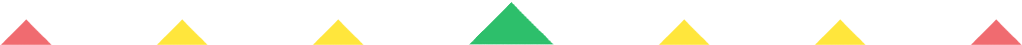Player 1
vs
Player 2
0:00
Opponent history
x
xCorrect
0
Wrong
0
Correct!
Wrong!
Help Sheet for

• 0 + 4 = 4
• 1 + 4 = 5
• 2 + 4 = 6
• 3 + 4 = 7
• 4 + 4 = 8
• 5 + 4 = 9
• 6 + 4 = 10
• 7 + 4 = 11